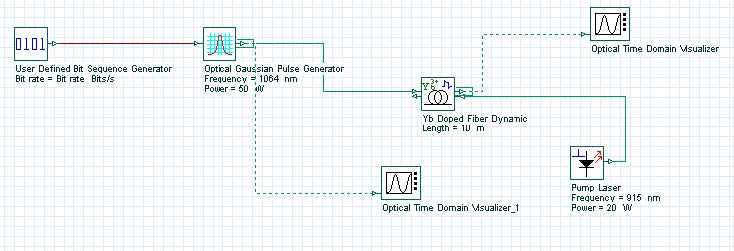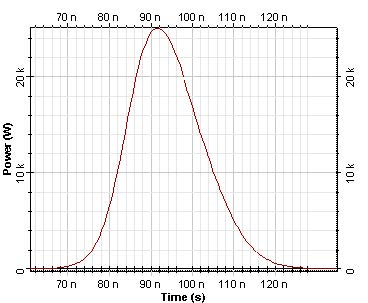The objective of this lesson is to demonstrate the amplification of a sequence of Gaussian pulses by an ytterbium-doped fiber amplifier using the dynamic model.

To analyze the dynamic characteristics of the high-power ytterbium-doped double-clad fiber amplifier considering pulses in repetition rates of KHz, a dynamic ytterbium-doped fiber model is necessary.

In this case, a sequence of 5 Gaussian pulses with a repetition rate of 100 KHz was used.

Figure 1 shows the system designed for this simulation. See sample Ytterbium_Double_Clad_Dynamic_100KHz.osd.Figure 1: System layout of the fiber amplifier

The input pulse peak power is 50 W and the energy in the pulse is 1uJ. The optical signal in the amplifier input with the sequence of Gaussian pulses is shown in Figure 2(a).

Figure 2: Optical signal at the amplifier (a) input and (b) output

The optical signal amplified is shown in Figure 2(b).

The first output pulse exceeds the successive ones because the energy stored in the amplifier was not recovered before the successive pulse.

The first output pulse can be seen in Figure 3.Figure 3: First output pulse